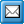﻿ Example Queries
QPR Knowledge Base 2017.1

Example QueriesExample QueriesThis chapter provides various examples of QPR Web Services Foundation usage. The "query:" and "attributes:" parts in these listing refer to parameters supplied to QueryObjects and QueryObjectsAsXml, and the "query:" and "attributes:" texts should not be included in the function calls.

Overlapping GetBinaryData and GetGraph functionality

With graphs GetBinaryData(<sessionid>, <objectId>, "graph(<options>)", <options>); returns similar results as GetGraph(<sessionid>, <objectId>, <options>);

Fetch the Actual and Target values for the latest ten periods for all scorecards with "Office" in their names

query:

@periodlevel = [SC.1938773693].PeriodLevel(criteria="Find(\"Quarter\", Name)");

@periods = @periodlevel.CurrentPeriod.PreviousPeriod(recursive=1, recursioncount=3, keeporiginals=1);

@scorecards = [SC.1938773693].Scorecard(criteria="Find(\"Office\", Name)");

@measures = @scorecards.TopElement.ChildObjects;

@measures

attributes:

scorecard.name, name, measure.values(series="ACT, TAR", periods=@periods)

Filter actions to show only those under johnsmith's responsibility

query:

[PO].Actions.Filter(responsible="johnsmith,UM.0.1")

Show all Comment and Risk actions with "Logistics" in their names

query:

[PO].Actions.Filter(include="Comment, Risk", criteria="Find(\"Logistics\", Name)")

Get actions connected to all elements with information items under [PG.1429039364] and its child levels

query:

@processlevels = [PG.1429039364].HierarchyRoots(hierarchy="process levels").ChildObjects(hierarchy="processlevels", recursive=1, keeporiginals=1);

@processlevelelements = @processlevels.SubObjects(keeporiginals=1);

@ = @processlevelelements.filter(include="\"Information item\"").ChildObjects(hierarchy="information items", recursive=1, keeporiginals=1);

Get all periods with measure values lower than 7 for the Actual period of Internal Processes measure of USA Sales Office

query:

@measure = [SC.1938773693.28];

@periodlevel = @measure.periodlevel;

@periods = @periodlevel.periods(criteriaroot="@measure", criteria="Measure.Value(period=\"@_\", series=\"ACT\") < 7");

@measure;

attributes:

name, measure.values(series="ACT", periods="@periods")

Returns all actions under the Discussion Forum in Portal

query:

[PO].HierarchyRoots(hierarchy="discussion").Related(hierarchy="discussion", keeporiginals="true", recursive="true")

attributes:

name, related(hierarchy="discussion")

Get all PG models

query:

[PG].HierarchyRoots(hierarchy="models").Related(hierarchy="models", keeporiginals="true", recursive="true")

attributes:

name, related(hierarchy="models")

Get all SC models

query:

[SC].HierarchyRoots(hierarchy="models").Related(hierarchy="models", keeporiginals="true", recursive="true")

attributes:

name, related(hierarchy="models")

Get all diagrams in the Dentorex - Quality Management System model

query:

[PG.1429039364].HierarchyRoots(hierarchy="processlevels").Related(hierarchy="processlevels", keeporiginals="true", recursive="true");

attributes:

name, related(hierarchy="processlevels")

Get all measures in the USA Sales Office scorecard in the Dentorex Group Scorecard model

query:

[SC.1938773693.21].HierarchyRoots(hierarchy="measures").Related(hierarchy="measures", keeporiginals="true", recursive="true")

attributes:

name, related(hierarchy="measures")

Find all subobjects with "The" in the name for all objects

query:

[PO, UM, SC, PG].Models(KeepOriginals=true).SubObjects(Criteria="Find(\"The\", Name)")

Find all actions under the responsibility of all users except for the default qpr/demo user ([UM.0.1])

query:

@users = [UM].Users;

@users -= [UM.0.1];

[PO].Actions.Filter(responsible="@users");